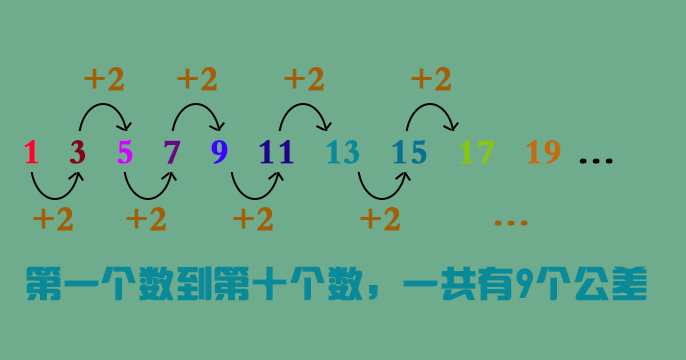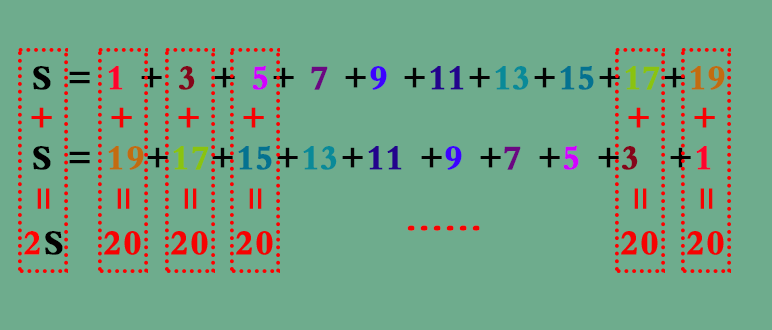# 1公差有什么用？

=(第八个数+2) +2=第八个数 +2×2

=(第七个数+2) +2×2=第七个数 +3×2

=(第六个数+2) +3×2=第六个数 +4×2

......

=(第二个数+2) +7×2=第二个数 +8×2

=(第一个数+2) +8×2=第一个数 +9×2

=1+9×2=192等差数列怎么求和？

B=6+5+4+3+2+1

（1）1+3+5+7+9+...+19 = 前十个数之和

（2）19+...+9+7+5+3+1 = 前十个数之和Sn=(a+b)×n÷2，b是第n项，b=a+(n-1)×d

• 电话咨询

400-8821-211
• 关注我们